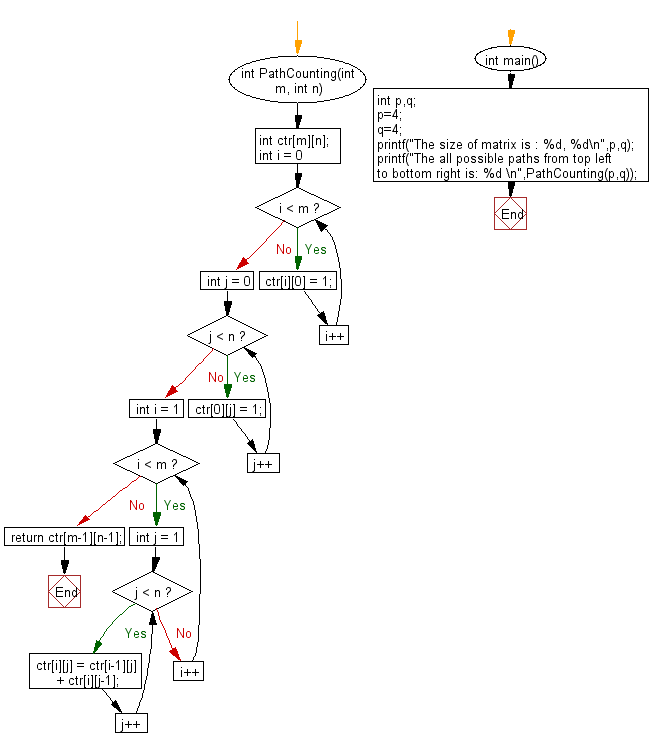﻿ C exercises: Count all possible paths from top left to bottom right of a m X n matrix - w3resource# C Exercises: Count all possible paths from top left to bottom right of a m X n matrix

## C Array: Exercise-85 with Solution

Write a program in C to Count all possible paths from top left to bottom right of a m X n matrix.

Sample Solution:

C Code:

``````#include <stdio.h>

int PathCounting(int m, int n)
{
int ctr[m][n];
for (int i = 0; i < m; i++)
{
ctr[i] = 1;
}
for (int j = 0; j < n; j++)
{
ctr[j] = 1;
}
for (int i = 1; i < m; i++)
{
for (int j = 1; j < n; j++)
{
ctr[i][j] = ctr[i-1][j] + ctr[i][j-1];
}
}
return ctr[m-1][n-1];
}

int main()
{
int p,q;
p=4;
q=4;
printf("The size of matrix is : %d, %d\n",p,q);
printf("The all possible paths from top left to bottom right is: %d \n",PathCounting(p,q));
}
```
```

Sample Output:

```The size of matrix is : 4 x 4
The all possible paths from top left to bottom right is: 20
```

Pictorial Presentation:Flowchart:C Programming Code Editor:

Improve this sample solution and post your code through Disqus.

﻿